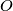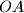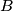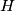IMO Shortlist 2017 problem G3

Kvaliteta:
Avg: 0,0
Težina:
Avg: 7,0

Let$O$ be the circumcenter of an acute triangle$ABC$. Line$OA$ intersects the altitudes of$ABC$ through$B$ and$C$ at$P$ and$Q$, respectively. The altitudes meet at$H$. Prove that the circumcenter of triangle$PQH$ lies on a median of triangle$ABC$.

Izvor: https://www.imo-official.org/problems/IMO2017SL.pdf